Courses

# Galvanic Cell - Electrochemistry Chemistry Notes | EduRev

## Chemistry : Galvanic Cell - Electrochemistry Chemistry Notes | EduRev

The document Galvanic Cell - Electrochemistry Chemistry Notes | EduRev is a part of the Chemistry Course Physical Chemistry.
All you need of Chemistry at this link: Chemistry

GALVANIC CELL

This cell converts chemical energy into electrical energy.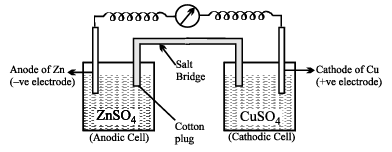Galvanic cell is made up of two half cells i.e., anodic and cathodic. The cell reaction is of redox kind.
Oxidation takes place at anode and reduction at cathode. It is also known as voltaic cell.  It may be represented as shown in Fig. Zinc rod immersed in ZnSO4 behaves as anode and copper rod immersed in CuSO4 behaves as cathode.

Oxidation takes place at anode:

Zn → Zn2+  + 2e-  (loss of electron : oxidation)

Reduction takes place at cathode:
Cu2+ + 2e →  Cu (gain of electron ; reduction)

Over all process:
Zn(s) + Cu2+     →   Cu(s) + Zn2+
In galvanic cell like Daniell cell; electrons flow from anode (zinc rod) to the cathode (copper rod) through external circuit; zinc dissolves as Zn2+ ; Cu2+ ion in the cathode cell picks up two electron and become deposited at cathode.

SALT BRIDGE

Two electrolyte solutions in galvanic cells are seperated using salt bridge as represented in the Fig.  salt bridge is a device to minimize or eliminate the liquid junction potential. Saturated solution of salt like KCI, KNO3, NH4Cl and NH4NO3 etc. in agar-agar gel is used in salt bridge. Salt bridge contains high concentration of ions viz. Kand NO3- at the junction with electrolyte solution. Thus, salt bridge carries whole of the  current across the boundary ; more over the K+and NO3- ions have same speed.
Hence, salt bridge with uniform and same mobility of cations and anions minimize the liquid junction potential & completes the electrical circuit  & permits the ions to migrate

REPRESENTATION OF AN ELECTROCHEMICAL CELL (GALVANIC CELL)

The following universally accepted conversions are followed in representing an electrochemical cell :

(i) The anode (negative electrode) is written on the left hand side and cathode (positive electrode) on the right hand side.
(ii) A vertical line or semicolon (;) indicates a contact between two phases. The anode of the cell is represented by writing metal first and then the metal ion present in the electrolytic solution.  Both are separated by a vertical line or a semicolon. For example Zn | Zn2+  or  Zn ; Zn2+ The molar concentration or activity of the solution is written in brackets after the formula of the ion.
For example

Zn | Zn2+  (1 M)  or  Zn | Zn2+ (0.1 M)

(iii) The cathode of the cell is represented by writing the cation of the electrolyte first and then metal. Both are separated by a vertical line or semicolon.
For example,

Cu2+ | Cu or Cu2+ ; Cu or Cu2+ (1 M) | Cu

(iv) The salts bridge which separates the two half-cells is indicated by two parallel vertical lines.

(v) Sometimes negative and positive signs are also put on the electrodes.
The Daniel cell can be represented as :

Zn | ZnSO4(aq)  ||  CuSO4(aq) | Cu

Anode        Salt bridge       Cathode

Oxidation    half-cellReduction half-cell

or Z n | Z n2+ || Cu2+ | Cu
or Zn | Zn2+ (1 M) || Cu2+ (1 M) | Cu

ELECTROCHEMICAL SERIES

By measuring the potentials of various electrodes versus standard hydrogen electrode (SHE), a series of standard electrode potentials has been established.  When the electrodes metals and non-metals) in contact with their ions are arranged on the basis of the values of their standard reduction potential or standard oxidation potential, the resulting series is called the electrochemical or electromotive or active series of the elements Standard Aqueous Electrode Potentials at 25°C

‘The Electrochemical Series’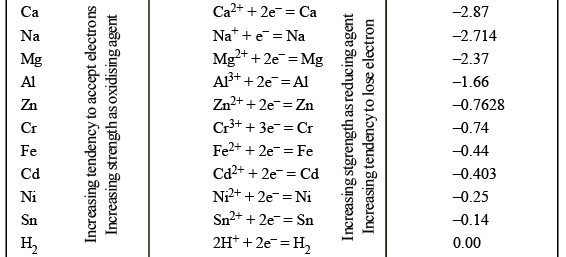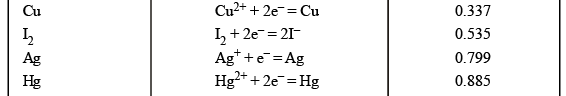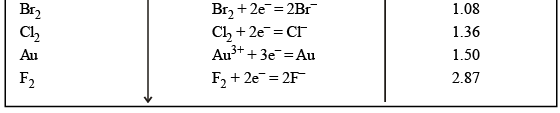APPLICATION  OF ELECTROCHEMICAL SERIES

(i) Reactivity of metals : The activity of the metal depends on its tendency to lose electron or electrons, i.e., tendency to form cation (Mn+).  This tendency depends on the magnitude of standard reduction potential. The metal which has high negative value (or smaller positive value) of standard reduction potential readily loses the electrons and is converted into cation.  Such a metal is said to be chemically active.
The chemical reactivity of metals decreases from top to bottom in the series. The metal higher in the series is more active than the metal lower in the series. For example,

(a) Alkali metals and alkaline earth metals having high negative values of standard reduction potentials are chemically active.  These react with cold water and evolve hydrogen. These readily dissolve in acids forming corresponding salts and combine with those substance which accept electrons.

(b) Metals like Fe, Pb, Sn, Ni, Co, etc., which lie a little down in the series do not react with cold water but react with steam to evolve hydrogen.

(c) Metals like Cu, Ag and Au which lie below hydrogen are less reactive and do not evolve hydrogen from water.

(ii) Electropositive character of metals : the electropositive character also depends on the tendency to lose electron or electrons. Like reactivity, the electropositive character of metals decreases from top to bottom in the electrochemical series. On the basis of standard reduction potential values, metals are divided into three groups :

(a) Strongly electropositive metals : Metals having standard reduction potential near about 2.0 volt or more negative like alkali metals, alkaline earth metals are strongly electropositive in nature.

(b) Moderately electropositive metals. Metals having values of reduction potential between 0.0 and about – 2.0 volt are moderately electropositive. Al, Zn, Fe, Ni, Co, etc., belong to this group.

(c) Weakly electropositive metals :  The metals which are below hydrogen and possess positive values of reduction potentials are weakly electropositive metals. Cu, Hg, Ag, etc., belong to this group.

(iii) Displacement reactions :

(a) To predict whether a given metal will displace another, from its salt solution :  A metal higher in the series will displace the metal from its solution which is lower in the series, i.e., the metal having low standard reduction potential will displace the metal from its salt’s solution which has higher value of standard reduction potential. A metal higher in the series has greater tendency to provide electrons to the cations of the metal to be precipitated.

(b) Displacement of one non-metals from its salt solution by another non metal : A nonmetal higher in the series (towards bottom side), i.e.,  having high value of reduction potential will displace another non metal with lower reduction potential i.e., occupying position above in he series. The nonmetal’s which possess high positive  reduction potentials have the tendency to accept electrons readily.  These electrons are provided by the ions of the nonmetal having low value of reduction potential.  Thus, Clcan displace bromine and iodine from bromides and iodides.

Cl2 + 2KI   →  2KCl + I2
2I- →  I2 + 2e- (Oxidation)
Cl+ 2e- →  2Cl- (Reduction)

[The activity or electronegative character or oxidising nature of the nonmetal increases as the value of reduction potential increases.]

(c) Displacement of hydrogen from dilute acids by metals :  The metal which can provide electrons to H+ ions present in dilute acids for reduction, evolve hydrogen from dilute acids.
Mn   →  Mnn+ + ne- (Oxidation)
2H+ 2e- →  H2 (Reduction)
The metal having negative values of reduction potential possess the property of losing electron or electrons.
Thus, the metals occupying top positions in the electrochemical series readily liberate hydrogen from dilute acids and on descending in the series tendency to liberate hydrogen gas from dilute acids decreases.
The metals which are below hydrogen in electrchemical series like Cu, Hg, Au, Pt, etc., do not evolve hydrogen from dilute acids.

(d) Displacement of hydrogen from water :  Iron and the metals above iron are capable of liberating hydrogen from water.  The tendency decreases from top to bottom in electrochemical series.
Alkali and alkaline earth metals liberate hydrogen from cold water but Mg, Zn and Fe liberate hydrogen from hot water or steam.

(iv) Reducing power of metals :  Reducing nature depends on the tendency of losing electron or electrons.
More the negative reduction potential, more is the tendency to lose electron or electrons. Thus, reducing nature decreases from top to bottom in the electrochemical series.  The power of the reducing agent increases as the standard reduction potential becomes more and more negative.
Sodium is a stronger reducing agent than zinc and zinc is a stronger reducing agent than iron.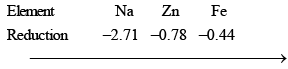Reducing nature decreases

Alkali and alkaline earth metals are strong reducing decreases.

(v) Oxidising nature of nonmetals : Oxidising nature depends on the tendency to accept electron of electrons. More the value of reduction potential, higher is the tendency to accept electron or electrons.
Thus, oxidising nature increases from top to bottom in the electrochemical series. The strength of an oxidising agent increases as the value of reduction potential becomes more and more positive.
F2 (Fluorine) is a stronger oxidant than Cl2 , Br2 and I2.
Cl2 (Chlorine) is a stronger oxidant than Br2 and I2.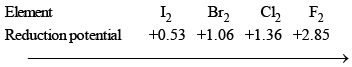Oxidising nature increases

Thus, in electrochemical series T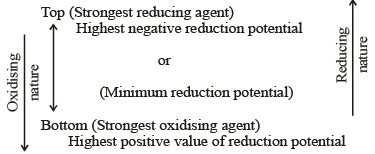(vi) Thermal stability of metallic oxides : The thermal stability of the metal oxide depends on its electropositive nature.  As the electropositivity decreases from to top bottom, the thermal stability of the oxide also decreases from top to bottom. The oxides of metals having high positive reduction potential are not stable towards heat.  The metals which come below copper form unstable oxides, i.e. these are decomposed on heating.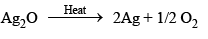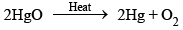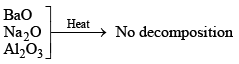(vii) Product of electrolysis : In case two or more type of positive and negative ions are present in solution, during electrolysis certain ions are discharged or liberated at the electrode in preference to others. In general, in such competition the ions which is stronger oxidising agent (high value of standard reduction potential) is discharged first at the cathode. The increasing order of deposition of few cations is :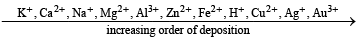Similarly, the anion which is stronger reducing agent (low value of standard reduction potential) is liberated first at the anode.
The increasing order of discharge of few anion is :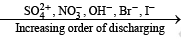Thus, when an aqueous solution of NaCl containing Na+, Cl, H+ and OH ions is electrolysed, H+ ions are discharged at cathode and Cl ions at the anode, i.e., H2 is liberated at cathode and chlorine at anode.
When an aqueous solution of CuSO4 containing Cu2+, SO42–, H+ and OH ions is electrolysed, Cu2+ ions are discharged at cathode and OH ions at the anode.
Cu2+ + 2e →  Cu (Cathodic reaction)
4OH →  O2 + 2H2O + 4e- (Anodic reaction)
Cu is deposited on cathode while O2 is liberated at anode.

(viii) Corrosion of metals :  Corrosion is defined as the deterioration of a substance because of its reaction with its environment.  This is also defined as the process by which metals have the tendency to go back to their combined state, i.e., reverse of extraction of metals.
Ordinary corrosion is a redox reaction by which metals are oxidised by oxygen in presence of moisture.
Oxidation of metals occurs more readily at points of strain.  Thus, a steel nail first corrodes at the tip and head. The end of a steel nail acts as an anode where iron is oxidised to Fe2+ ions.
Fe   →  Fe2 + 2e-  (Anode reaction)
The electrons flow along the nail to areas containing impurities which act as cathodes where oxygen is reduced to hydroxyl ions.
O2 + 2H2O + 4e →  4OH-  (Cathode reaction)

REVERSIBLE AND IRREVERSIBLE CELLS

In dealing with the energy relations of cells, thermodynamic principles find extensive applications.
However, the use of these principles is subject to one important restriction, namely, that the system to which they are applied be reversible in thermodynamic sense.  This require that :
(1) the driving and opposing forces the infinitesimally different from each other, and
(2) it should be possible to reverse any change taking place by applying a force infinitesimally greater than the acting one.
A cell satisfying the above two requirements constitutes a reversible cell.  The potential difference of the cell can be substituted into the relevant thermodynamic relations and hence the value of thermodynamic properties such as free energy change, entropy change and enthalpy change of the cell reaction can be determined.  When the above conditions are not satisfied, the cell is said to be irreversible, and thermodynamic relations do not apply.
The difference between reversible and irreversible cells may be illustrated with the following two examples.

Example of Reversible Cell

Consider a cell composed of Zn and Ag-AgCl electrodes into an aqueous solution of zinc chloride. As seen earlier, the following reactions take place on connecting externally.
Anode 1/2 Zn(s) →  1/2 Zn2+(aq) + e-
Cathode : AgCl(s) + e- → Ag(s) + Cl-(aq)
with the net reaction 1/2Zn(s) + AgCl(s) → Ag(s) + 1/2 Zn2+(aq) + Cl-(aq)

The above process continues as long as the external opposing potential is infinitesimally smaller than that of the cell.  However, if the opposing potential becomes slightly larger than that of the cell, the direction of current flow is reversed, and so is the cell reaction.  Now zinc ions are converted to zinc at one electrode, silver chloride is formed from silver and chloride ions at the other, and the overall cell reaction becomes

Ag(s) 1/2 Zn2+ (aq) + Cl-(aq) → 1/2 Zn(s) + AgCl(s)
Thus, it is  obvious that the second condition of irreversibility mentioned above is satisfied.  The first condition can be satisfied by drawing from or passing through the cell a very minute current. Hence, the cell is reversible.

STANDARD ELECTRODE POTENTIAL

The potential difference developed between metal electrode and the solution of its ions of unit molarity (1 M) at 25°C (298 K) is called standard electrode potential.

Standard reduction potential = – (Standard oxidation potential)
or Standard oxidation potential = – (Standard reduction potential)

CONCEPT OF ELECTROMOTIVE FORCE (EMF) OFA CELL

Electron flows from anode to cathode in external circuit due to a pushing effect called electromotive force (e.m.f.). E.m.f. is some times called as cell potential. Unit of e.m.f. of cell is volt.
EMF of cell may be calculated as :
Ecell = reduction potential of cathode  - Reduction potential of anode
Similarly, standard e.m.f. of the cell (E°) may be calculated as
cell  = Standard reduction potential  of cathode - Standard reduction potential of anode

SIGN CONVENTION OF EMF
EMF of cell should be positive other wise it will not be feasible in the given direction .
Zn | ZnSO4 || CuSO4 | Cu        E = +1.10 volt (Feasible)
Cu | CuSO4 || ZnSO4 | Zn           E = -1.10 volt  (Not Feasible)

Nernst Equation

• For a single electrode involving the reduction process, Mn+ + ne- → M, the nernst equation is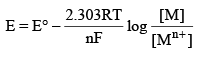or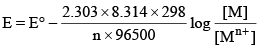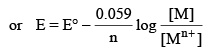•  For an electrochemical cell having net reaction :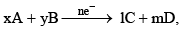the EMF can be calculated as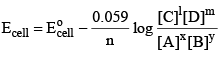•  In using the above equation, the following facts should be kept in mind.
(i) Concentration or activity of solids is taken to be UNITY.
(ii) Concentration of activity of gases is expressed in terms of their partially pressures.
(iii) n, the number of electrons transferred should be calculated from the balanced net reaction.

Note :

• Relationship between free energy change and cell potential can be written as
ΔG = -nF Ecell
For standard state condition
ΔG° = -nF E°cell
• Equilibrium constant of net cell reaction is related to the standard EMF as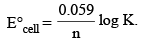EMF  OF GALVANIC CELL

Every galvanic or voltaic cell is made up of two half-cells, the oxidation half-cell (anode) and the reduction half-cell (cathode). The potential of these half-cells are always different. On account of this difference in electrode potentials, the electric current moves from the electrode at higher potential to the electrode at lower potential, i.e. from cathode to anode. The direction of the flow of electrons is from anode to cathode.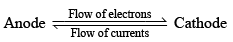The difference in potential of the two half-cells is known as the electromotive force (emf) of the cell or cell potential.

Offer running on EduRev: Apply code STAYHOME200 to get INR 200 off on our premium plan EduRev Infinity!

## Physical Chemistry

66 videos|94 docs|32 tests

,

,

,

,

,

,

,

,

,

,

,

,

,

,

,

,

,

,

,

,

,

;